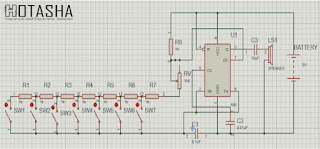# How to Make a Electronic Piano Using NE555 Timer

A simple electronic toy piano can be easily created using the popular 555 timer IC. The 555 timer can be easily wired as a stable, nonstable, beatable, multi vibrator.

We know that sound is a mechanical wave because it is produced by the vibrations of particles in the medium. The displacement of particles and the propagation of sound waves are in the same direction so the sound is a longitudinal wave. The medium particles create contraction and expansion between the two and the flow motion medium, so the sound is a pressure wave.Loudspeaker and sound reception

A melodious sound is produced by regular vibrations of its source. So it has only one frequency though the intensity / amplitude may vary. Loudspeaker is an electronic transducer. It has a screen on the surface, which makes different vibrations for different vibrations. This screen converts electrical signals into pressure changes to create sound sensations. The loudspeaker will vibrate according to the frequency and amplitude of the electrical signal to create this diaphragm.

The human audible frequency range is from 20Hz to 20KHz. So we're going to create a frequency in this range using the 555 timer and send it to the loudspeaker and listen in the form of sound. And only if we can bring a regular relationship to the vibration of this sound will that sound be melodious. So let's get started-

Necessary materials

• 555 Timer IC – 1.
• Speaker 8Ω, 0.5W – 1.
• Resistor 1K – 8.
• Variable Resistor 10K-1.
• Electrolytic Capacitor 10μF-1.
• Ceramic Capacitor 0.1μF-1.
• Ceramic Capacitor 0.01μF-1.
• Push Button Switch-7.Circuit image

Circuit diagram

Once the materials are collected, they need to be connected to the breadboard like the circuit in the picture. If you can make it on the breadboard without connecting to the PCB or Vero board first, then there will be no problem to place it on the PCB or Vero board later.

Here,

If you know the work of a stable multivibrator using 555, we can easily understand the work of the above circuit. Capacitor C1 (0.1μF) and all the resistors in the upper circuit determine the oscillation frequency of the multivibrator. Capacitor C2 (0.01μF) is connected to the 5th pin to avoid high frequency and capacitor C3 (10μF) acts as a coupling capacitor that blocks the AC signals in the loudspeaker. By changing the variable resistor Arabic Rx (10 kΩ) we can change the generated tones.

Using 555 the output frequency that the equation adheres to,

F = 1.44 / (R_9 + 2RV) C1.

Where R9 and C1 have values ​​of 1KΩ and 0.1 মানF respectively, the value of RV is determined by the pressure switch-

• When SW1 is pressed, RV = R1 + R2 + R3 + R4 + R5 + R6 + R7
• When SW2 is pressed, RV = R2 + R3 + R4 + R5 + R6 + R7
• When SW3 is pressed, RV = R3 + R4 + R5 + R6 + R7
• When SW4 is pressed, RV = R4 + R5 + R6 + R7
• When SW5 is pressed, RV = R5 + R6 + R7
• When SW6 is pressed, RV = R6 + R7
• When SW7 is pressed, RV = R7

Thus pressing each switch will generate a similar frequency, through which the speaker will generate different tones (single frequency sound) for each switch.

We can operate 555 IC from a power supply of 4.5 to 18 volts, but it should not exceed the power rating of the loudspeaker.

Caution

• The power supply must be between 7 and 18 volts in the circuit. Even if the piano is played less, the sound will be less or not. However, if it is more than 16 volts, the IC will burn out.
• Avoid loose connections.
• 6 In case of PCB connection, care should be taken not to exceed the temperature rating of IC.
• 6 In case of capacitor connection, the connection should be given by looking at the positive and negative.

What to do if the connection does not work

• See if there are any loose connections.
• Make sure the IC is OK. If necessary, we have to change it.
• 6 See if the power supply is within range.
• 6 If necessary, you can see from the multimeter whether all the resistors, capacitors and switches are working properly.

Edited By
Jeion Ahmed
B.Sc. in Electrical & Electronic Engineering (EEE)
Chittagong University of Engineering & Technology (CUET)